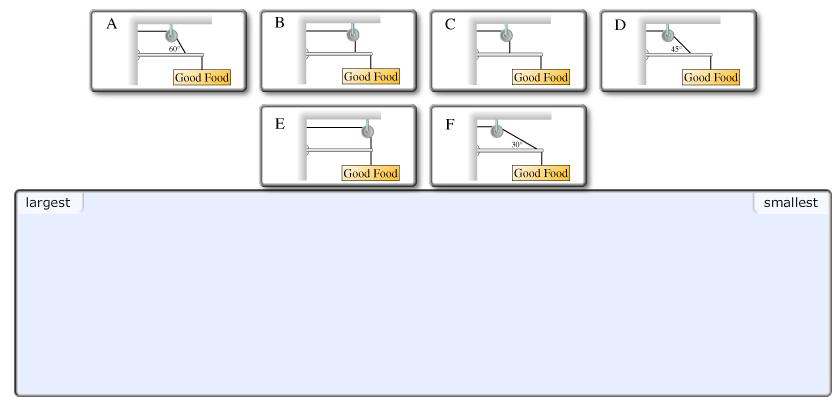# Problem: A sign is to be hung from the end of a thin pole of length L, and the pole supported by a single cable. Your design firm brainstorms the six scenarios shown below. In scenarios A, B, and D, the cable is attached halfway between the midpoint and end of the pole. In C, the cable is attached to the mid-point of the pole. In E and F, the cable is attached to the end of the pole.a. Rank the design scenarios (A through F) on the basis of the tension in the supporting cable.b. Rank from largest to smallest. To rank items as equivalent, overlap them.

###### FREE Expert Solution

The expression for the tension:

$\overline{)\begin{array}{rcl}\mathbf{r}\mathbf{T}\mathbf{s}\mathbf{i}\mathbf{n}\mathbf{\theta }& {\mathbf{=}}& \mathbf{L}\mathbf{W}\\ {\mathbf{T}}& {\mathbf{=}}& \frac{\mathbf{L}\mathbf{W}}{\mathbf{r}\mathbf{s}\mathbf{i}\mathbf{n}\mathbf{\theta }}\end{array}}$

For A:

$\begin{array}{rcl}{\mathbf{T}}_{\mathbf{A}}& \mathbf{=}& \frac{\mathbf{\left(}\mathbf{8}\mathbf{.}\mathbf{00}\mathbf{\right)}\mathbf{W}}{\mathbf{\left(}\mathbf{3}\mathbf{/}\mathbf{4}\mathbf{\right)}\mathbf{sin}\mathbf{60}}\end{array}$

TA = 12.32W N

For B:

$\begin{array}{rcl}{\mathbf{T}}_{\mathbf{B}}& \mathbf{=}& \frac{\mathbf{\left(}\mathbf{4}\mathbf{.}\mathbf{00}\mathbf{\right)}\mathbf{W}}{\mathbf{\left(}\mathbf{3}\mathbf{/}\mathbf{4}\mathbf{\right)}\mathbf{sin}\mathbf{90}}\end{array}$

TB = 5.33W N

100% (59 ratings)###### Problem Details

A sign is to be hung from the end of a thin pole of length L, and the pole supported by a single cable. Your design firm brainstorms the six scenarios shown below. In scenarios A, B, and D, the cable is attached halfway between the midpoint and end of the pole. In C, the cable is attached to the mid-point of the pole. In E and F, the cable is attached to the end of the pole.a. Rank the design scenarios (A through F) on the basis of the tension in the supporting cable.

b. Rank from largest to smallest. To rank items as equivalent, overlap them.